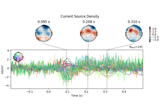# mne.preprocessing.compute_current_source_density#

mne.preprocessing.compute_current_source_density(inst, sphere='auto', lambda2=1e-05, stiffness=4, n_legendre_terms=50, copy=True, *, verbose=None)[source]#

Get the current source density (CSD) transformation.

Transformation based on spherical spline surface Laplacian .

This function can be used to re-reference the signal using a Laplacian (LAP) “reference-free” transformation.

Parameters:
instinstance of `Raw`, `Epochs` or `Evoked`

The data to be transformed.

spherearray-like, shape (4,) | `str`

The sphere, head-model of the form (x, y, z, r) where x, y, z is the center of the sphere and r is the radius in meters. Can also be “auto” to use a digitization-based fit.

lambda2`float`

Regularization parameter, produces smoothness. Defaults to 1e-5.

stiffness`float`

Stiffness of the spline.

n_legendre_terms`int`

Number of Legendre terms to evaluate.

copybool

Whether to overwrite instance data or create a copy.

verbose

Control verbosity of the logging output. If `None`, use the default verbosity level. See the logging documentation and `mne.verbose()` for details. Should only be passed as a keyword argument.

Returns:
inst_csdinstance of `Raw`, `Epochs` or `Evoked`

The transformed data. Output type will match input type.

Notes

New in version 0.20.

References

## Examples using `mne.preprocessing.compute_current_source_density`#Transform EEG data using current source density (CSD)

Transform EEG data using current source density (CSD)